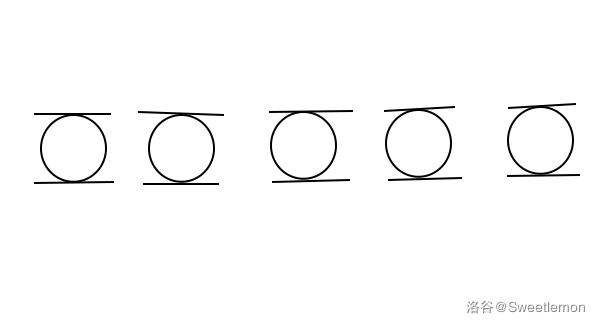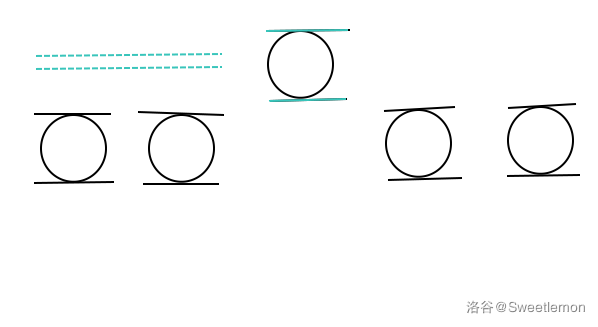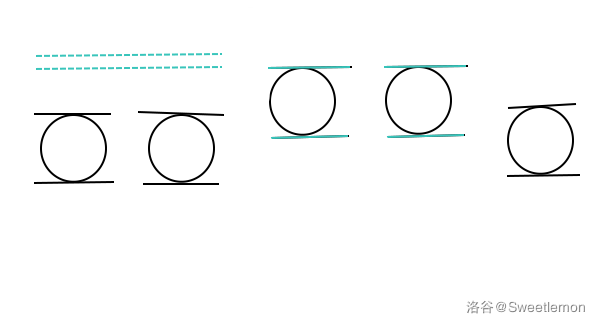# Sweetlemon 的博客# Sweetlemon 的博客

### 区间与球

posted on 2019-11-04 09:31:15 | under 题解 |

### [IOI2014] Wall 砖墙

#### 题意

1. 对某区间内的所有数 $x$ ，令 $x=\max(t,x)$ （ $t$ 是操作参数）
2. 对某区间内的所有数 $x$ ，令 $x=\min(t,x)$

#### 思路

“萌萌哒”这道题中，操作之间互不影响，而且操作区间可以重叠，因此可以直接使用 st 表（若操作区间不可重叠，同样可使用 st 表，但操作区间要被二进制拆分成为 $O(\log l)$ 个）。但是在这道题中，不同的操作前后会有影响，因此我们可以使用线段树。

5 2
1 1 3 2
2 3 4 1#### 代码

#include <cstdio>
#include <cctype>
#include <algorithm>
#define MAXIOLG 25
#define MAXN 2000005
#define MAX4N 8000005
#define INF 19260817
using namespace std;

typedef int io_t;

//快读快写
io_t shin[MAXIOLG];
io_t seto(void);
void ayano(io_t x,char spliter='\n');

//线段树节点
struct Ene{
int l,r,mxm,mnm;
//以下依次为节点区间左端点、右端点、m 值、n 值、左右儿子序号
#define L(x) ((stree[(x)]).l)
#define R(x) ((stree[(x)]).r)
#define MX(x) ((stree[(x)]).mxm)
#define MN(x) ((stree[(x)]).mnm)
#define LC(x) (((x)<<1))
#define RC(x) (((x)<<1)^1)
};

int n;
Ene stree[MAX4N]; //线段树

void build_tree(int root,int l,int r); //建树
void cmx(int root,int l,int r,int x); //1 操作
void cmn(int root,int l,int r,int x); //2 操作
void pushdown(int root); //标记下传,把父节点的操作应用到子节点
void output(int root); //输出答案

int main(void){
int q;
n=seto(),q=seto();
const int troot=1; //线段树根节点
build_tree(troot,1,n);
while (q--){
int op,l,r,x;
op=seto(),l=seto()+1,r=seto()+1,x=seto();
if (op==1)
cmx(troot,l,r,x);
else
cmn(troot,l,r,x);
}
output(troot);
return 0;
}

void build_tree(int root,int l,int r){
//建树
//初始时非叶节点没有限制
L(root)=l,R(root)=r,MX(root)=0,MN(root)=INF;
if (l==r)
//叶节点有限制, m=n=0
return MX(root)=MN(root)=0,void();
int mid=(l+r)>>1;
build_tree(LC(root),l,mid);
build_tree(RC(root),mid+1,r);
}

void cmx(int root,int l,int r,int x){
if (l<=L(root) && r>=R(root)){
//把限制标记在这个区间
//m,n 都取 max
MX(root)=max(MX(root),x);
MN(root)=max(MN(root),x);
return;
}
int mid=(L(root)+R(root))>>1;
pushdown(root);

(l<=mid)?(cmx(LC(root),l,r,x)):(void());
(r>mid)?(cmx(RC(root),l,r,x)):(void());
}

void cmn(int root,int l,int r,int x){
if (l<=L(root) && r>=R(root)){
//m,n 都取 min
MX(root)=min(MX(root),x),
MN(root)=min(MN(root),x);
return;
}
int mid=(L(root)+R(root))>>1;
pushdown(root);
(l<=mid)?(cmn(LC(root),l,r,x)):(void());
(r>mid)?(cmn(RC(root),l,r,x)):(void());
}
void pushdown(int root){
//标记下传
if (L(root)==R(root))
return;
//对左右儿子的 m,n 值都应用等效 2 操作
MX(LC(root))=min(MX(LC(root)),MN(root));
MX(RC(root))=min(MX(RC(root)),MN(root));
MN(LC(root))=min(MN(LC(root)),MN(root));
MN(RC(root))=min(MN(RC(root)),MN(root));
//对左右儿子的 m,n 值都应用等效 1 操作
MX(LC(root))=max(MX(LC(root)),MX(root));
MX(RC(root))=max(MX(RC(root)),MX(root));
MN(LC(root))=max(MN(LC(root)),MX(root));
MN(RC(root))=max(MN(RC(root)),MX(root));
//父节点的限制应用完毕，取消限制
MX(root)=0,MN(root)=INF;
}
void output(int root){
if (L(root)==R(root)){
ayano(MX(root));
return;
}
pushdown(root);
output(LC(root)),output(RC(root));
}

//以下两个函数为读入输出优化

io_t seto(void){
io_t x=0;
int symbol=0;
char ch=getchar();
while (!isdigit(ch))
(ch=='-')?(symbol=1):(0),
ch=getchar();
while (isdigit(ch))
x=(x*10)+(ch-'0'),
ch=getchar();
return (symbol)?(-x):(x);
}

void ayano(io_t x,char spliter){
if (!x){
putchar('0'),putchar(spliter);
return;
}
if (x<0)
putchar('-'),x=-x;
int len=0;
while (x){
io_t d=x/10;
shin[len++]=x-(d*10);
x=d;
}
while (len--)
putchar(shin[len]+'0');
putchar(spliter);
}